## COMP 256 Programming Assignment 2

Epipolar Geometry and Stereo. (assignment)

### Algorithm

To extract point correspondences, I wrote a program in Borland Delphi Personal Edition, a RAD programming environment (free for personal use). The image below shows a screenshot of the program.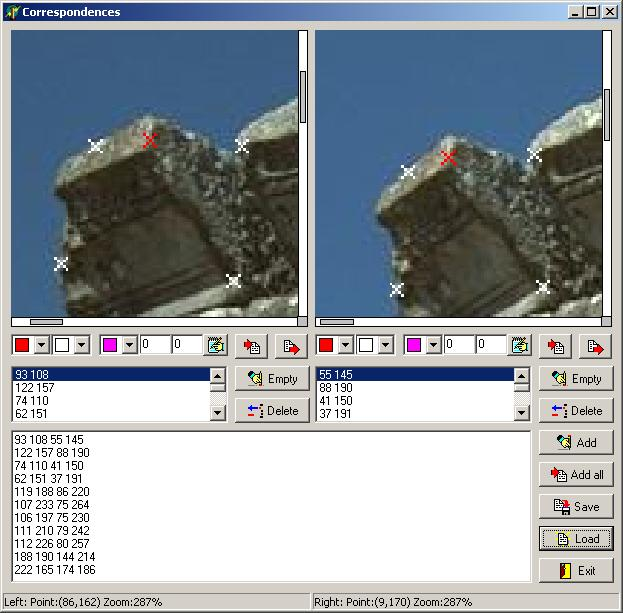It allowed me to easily get a relatively large number of correspondences. I used 59 correspondences for the algorithm.

Its features also include the ability to zoom in and out, add and delete correspondences, load and save them as a text file, as well as draw the epipolar lines if the locations of the epipoles are known.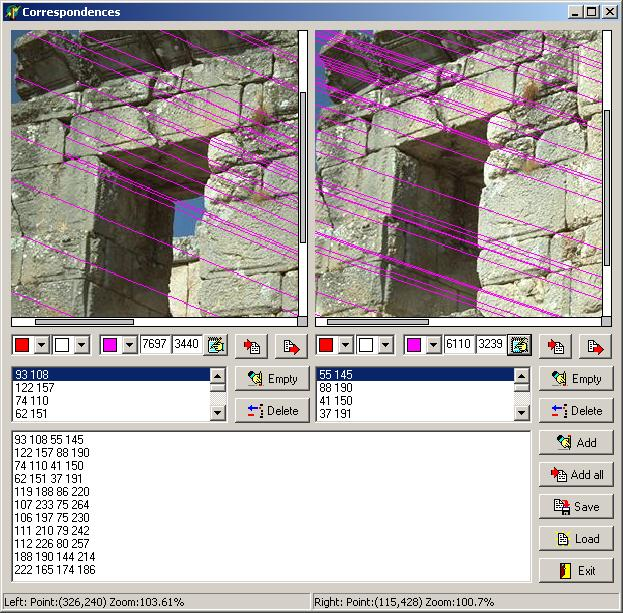I then proceeded to implement the 8 point algorithm on page 219 of the book. I first tried to use the anisotropic scaling method using Choleski factorization as suggested by Hartley in In Defense of the Eight-Point Algorithm, but the results were not as good as I expected. I reverted to isotropic scaling as recommended in the book.

The epipoles were at (7697,3440,1) and (-126110,-43239,1), and the epipolar lines are shown below:

To compute the rectifying homographies, I tried to use Hartley's approach from Theory and Practice of Projective Rectification. As it was not clear to me how to compute the second homography from the first, I just applied a modified version of the algorithm for each image:

• for each reference point (feature) Pi
• translate Pi and the epipole so that Pi is at the origin (Ti)
• rotate around Pi so that the epipole ends up on the x axis at point (f,0,1) (Ri)
• move the epipole to infinity (Ki=[1 0 0;0 1 0;-1/f 0 1])
• store the homography Wi=Ki*Ri*Ti
• compute the mean of all the stored homographies Wi

While computing the mean has no mathematical justification, the results were better than just picking the center of the image as the reference point.

The images warped using the computed homographies are shown below. The warped epipolar lines are parallel and horizontal (modulo the interpolation artifacts), and corresponding lines are at approximately the same height.

For computing the stereo correspondences, I implemented gray level matching using zero-mean normalized cross correlation on luminance values, similar to the approach presented here. Since the images computed in the warping process were not of the same size, I padded the smaller one and added an offset.

The algorighm consists of computing a 3D cross correlation matrix for a window of size (2K+1,2L+1), sliding one of the images with respect to the other. The result is a "cuboid" as the one shown below (figure taken from the page mentioned earlier):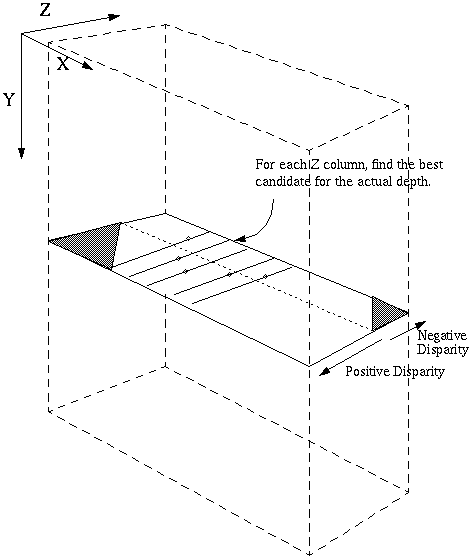The disparity image is then computed at each pixel as the index of the maximum normalized cross-correlation value for a particular point.

The formulas for computing the normalized cross correlation are as follows (figures taken from the page mentioned earlier):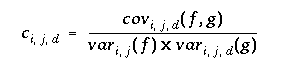where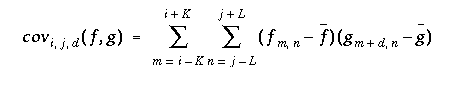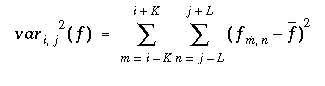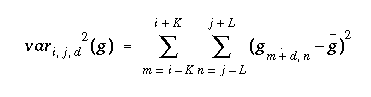To speed up the correlation computation, I precomputed the values of the mean luminance and variance over a neighborhood for both images.

I then experimented with different window sizes. The disparity images below are for windows of 11x11 and 21x21 pixels (only left-to-right disparity, no coherence enforced, saved using HDR Shop):

Finally, I enforced left-to-right and right-to-left coherence on the disparity images. The resulting disparity images are shown below (saved using HDR Shop):

To visually verify the stereo matching, I constructed the left image from the right viewpoint and the right image from the left viewpoint: